Finite Automata

# Finite Automata - Notes | Study Theory of Computation - Computer Science Engineering (CSE)

 1 Crore+ students have signed up on EduRev. Have you?

Part II. Finite Automata

Different classes of automata are there. examples: finite automata, pushdown automata. Of them finite automata is the simplest one.In Module I, during the discussion of chomsky classification, it was seen that type-3 or regular languages are recognised using finite automata.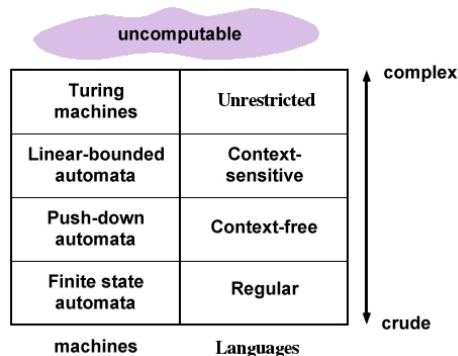Definition
A finite automation, M is a 5 tuple structure,
M ( Q, ∑, q0, δ, F )
where M is the finite automation,
Q is a finite set of states,
∑ is a set of input symbols,
q0 is the start state,
δ is the set of transition functions,
F is the set of final states.
Consider the following example for a finite automation, M.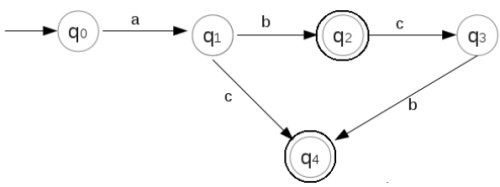In the above finite automation, M,
1. Q = {q0, q1, q2, q3, q4}
Q is the set of states in the finite automata. In the above finite automata, q0, q1, q2, q3, q4 are the states. The states are shown in circles.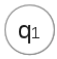2. ∑ = a, b, c
∑ is the set of input symbols in the finite automata. In the above, a, b, c are the input symbols. The arrowsare labelled with input symbols.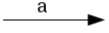3. q0 = {q0}
q0 is the start state. In the above, q0 is the start state. For our study, a finite automata contains only one start state. A state with arrow from free space (not coming from any other state) is designated as start state.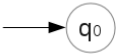4. δ describes the operation of finite automation. δ is the transition function.
For example,
δ(q0, a) = q1
This means, given the current state q0 and the input symbol, a, the finite automation moves (transits) to state q1.
Similarly,
δ(q1, b) = q2
δ(q2, c) = q3
δ(q1, c) = q4
δ(q3, b) = q4

5. F = {q2, q4}
F is the set of final states or accepting states. In the above finite automation, q and q4 are the final states. They are shown enclosed in double circles.

Transition Diagrams and Transition Tables
Consider the following finite automation, M.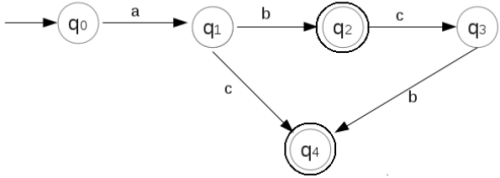The above representation of finite automation is called a transition diagram. A finite automata can also be represented as a transition table.This is shown below: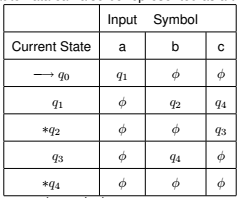→ q0 denotes that q0 is the start state.
∗ before q2 and q4 indicates that q2 and qare the final states.

3 Language Acceptibility by Finite Automata

String Processing by Finite Automation
Example 1:
Consider the following finite automation, M.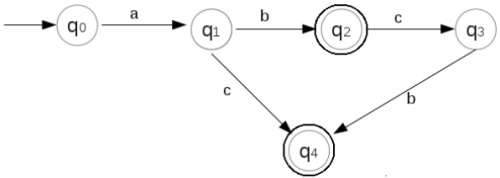Check whether the string abcb is accepted by the above finite automation.

In the above, q0 is the start state. the string abcb has the first symbol, a.
So,
δ(q0, abcb) = q1
δ(q1, abcb) = q2
δ(q2, abcb) = q3
δ(q3, abcb) = q4
Thus,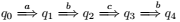Thus, after processing the string abcb, we reached the state, q4. Here q4 is a final state. So the string abcb is accepted by the finite automation.

Example 2:
Consider the following finite automation, M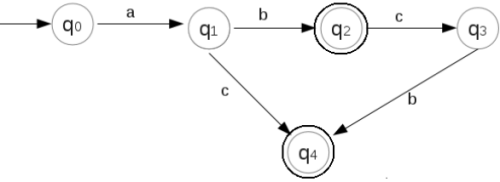Check whether the string abc is accepted by M.
On processing the string, beginning from the start state, q0,
δ(q0, abc) = q1
δ(q1, abc) = q2
δ(q2, abc) = q3
Here M halts at q3. But qis not a final state. Hence the string abc is rejected by the above finite automation, M.

Example 3:
Conisder the finite automation, M,Check whether the string abca is accepted by the finite automation, M.
Beginning from the start state, q0,
δ(q0abca) = q1
δ(q1, abca) = q2
δ(q2, abca) = q3
δ(q3, abca) =
Here for current state, q3, and for input symbol, a, there is no transition. This means M is unable to process the string abca completely. So the string abca is rejected by M.

Language of Finite Automation
In the above, finite automation is used to check the validity of a string. A language is a finite set of strings. So we can use
finite automation to check whether a string belongs to a language.
Consider the following finite automation,Let L be the language corresponding to the above finite automation, M. Then a string belongs to the language L only if it is accepted by the finite automation, M.
We can say that,
The string abcb belongs to the language, L.
The string abc does not belong to the language, L.
The string abca does not belong to the language, L.
The string ab belongs to the language, L.
The string ac belongs to the language, L.
The string abcbc does not belong to the language , L.

4 Types of Finite Automata
Finite automation is of different types. They are,
Non-deterministic Finite Automata (NFA), and
Deterministic Finite Automata (DFA).

4.1 Non- Deterministic Finite Automata (NFA)
Consider the following finite automation, M,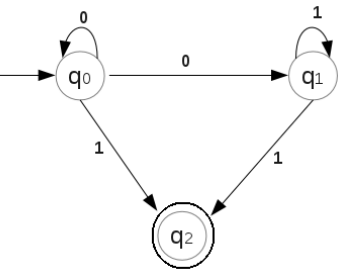In the above, from state qwith input symbol 0, there are two possible next states. The next state can be either q0 or q1.
Also from state q1, on input symbol, 1, there are two possible next states. The next state can be either q1or q2.
Thus some moves of the finite automation cannot be detrmined uniquely by the input symbol and the current state.
Such finite automation are called Non-deterministic Finite automata (NFA or NDFA).

Example 1:
Consider the following NFA,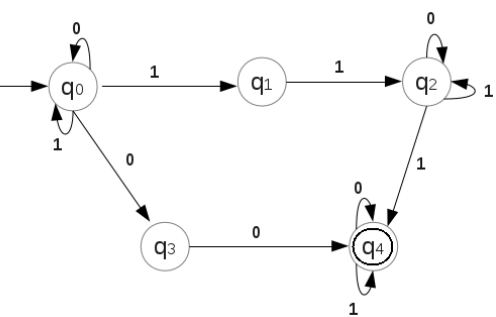Check whether the string 0100 is accepted by the above NFA.
Beginning from the start symbol, q0,
δ(q0, 0100) = q0
δ(q0, 0100) = q0
δ(q0, 0100) = q3
δ(q3, 0100) = q4
q4 is a final state. So the string 0100 is accepted by the above finite automation.

Example 2:
Consider the following NFA,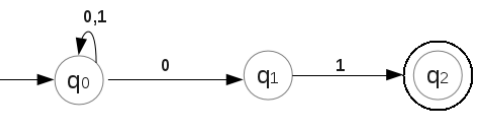Check whether the string 01101 is accepted by the above NFA.
δ(q0, 01101) = q0
δ(q0, 01101) = q0
δ(q0, 01101) = q0
δ(q0, 01101) = q1
δ(q1, 01101) = q2
q2 is a final state. So the string 01101 is accepted by the above NFA.

Example 3: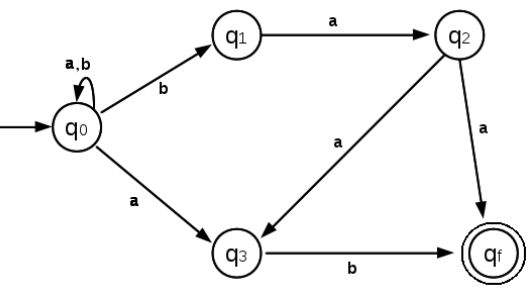Check whether the string abbaa is accepted by the above NFA.
δ(q0, abbaa) = q0
δ(q0, abbaa) = q0
δ(q0, abbaa) = q1
δ(q1, abbaa) = q2
δ(q1, abbaa) = qf
qf is a final state. So the string abbaa is accepted by the above NFA.

4.1.1 NFA with Epsilon Transitions

An NFA can contain certain transitions on input symbol, ε (epsilon).
ε means ’null’.
For example, consider the following NFA,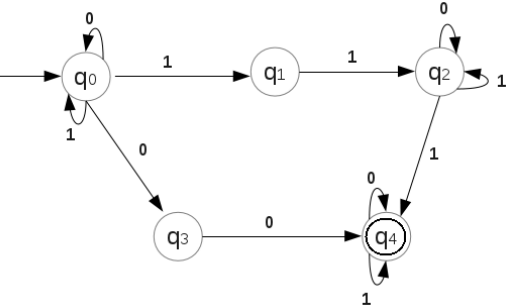Check whether the string 100110 is accepted by the above NFA.

δ(q0, 100110) = q0
δ(q0, 100110) =q0
δ(q0, 100110) = q0
δ(q0, 100110) = q1
δ(q1, 100110) = q2
δ(q2, 100110) = q2
δ(q2, ε) = q4
q4 is a final state. So the string 100110 is accepted by the above NFA.
Here note that, we made an ε move to reach the final state, q4.
Thus, ε transition means a transition without scanning the symbol in the input string.

Example 2:
Consider the following NFA,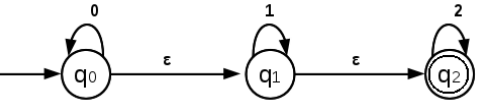Check whether the string 0012 is accepted by the above NFA.
Beginning with the start state, q0,
δ(q0, 0012) = q0
δ(q0, 0012) = q0
δ(q0, ε) = q1
δ(q1, 0012) = q1
δ(q1, ε) = q2
δ(q2, 0012) = q2
Here q2 is a final state. So the string 0012 is accepted by the NFA.

4.2 Deterministic Finite Automata
Consider the following finite automation,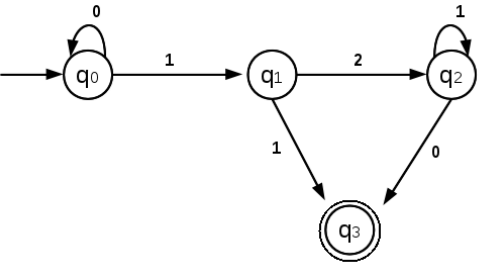In the above, input symbols are 0, 1 and 2. From every state, there is only one transition for an input symbol.
From state, q0, for symbol, 0, there is only one transition, that is to state q0 itself.
From state, q0, for symbol, 1, there is only one transition, that is to state q1.
From state, q1, for symbol, 1, there is only one transition, that is to state q3, and so on.
Thus all the moves of the above finite automation can be determined uniquely by the current state and input symbol.
Such a finite automation is called Deterministic finite Automation (DFA).
Thus in a DFA, at every step, next move is uniquely determined. No ε transitions are present in a DFA.

Example 1:
The following shows a DFA,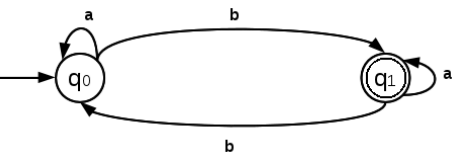Above transition diagram can be represented using the transition table as follows: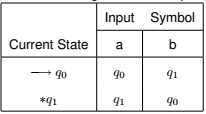Check whether the string aaba is accepted by the above DFA.
Beginning from the start state, q0,
δ(q0, aaba) = q0
δ(q0, aaba) = q0
δ(q0, aaba) = q1
δ(q1, aaba) = q1
After processing all the characters in the input string, final state qis reached. So the string aaba is accepted by the above finite automation.

Example 2:
Consider the following DFA,

Check whether the string aabba is accepted by the above DFA.
δ(q0, aabba) = q0
δ(q0, aabba) = q0
δ(q0, aabba) = q1
δ(q1, aabba) = q0
δ(q0, aabba) = q0
After processing all the symbols in the input string, aabba, the state q0 is reached. But q0 is not a final state. So the DFA rejects the string aabba.

The document Finite Automata - Notes | Study Theory of Computation - Computer Science Engineering (CSE) is a part of the Computer Science Engineering (CSE) Course Theory of Computation.
All you need of Computer Science Engineering (CSE) at this link: Computer Science Engineering (CSE)

## Theory of Computation

18 videos|56 docs|41 tests
 Use Code STAYHOME200 and get INR 200 additional OFF

## Theory of Computation

18 videos|56 docs|41 tests

Track your progress, build streaks, highlight & save important lessons and more!

,

,

,

,

,

,

,

,

,

,

,

,

,

,

,

,

,

,

,

,

,

;